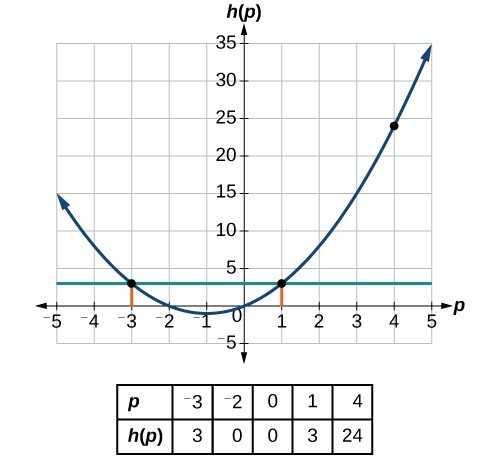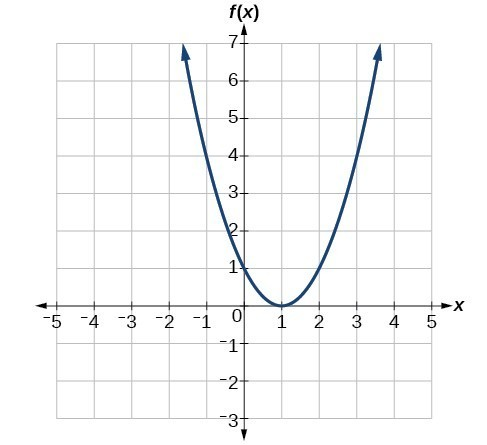## Find the input and output values of a function

When we know an input value and want to determine the corresponding output value for a function, we evaluate the function. Evaluating will always produce one result because each input value of a function corresponds to exactly one output value.

When we know an output value and want to determine the input values that would produce that output value, we set the output equal to the function’s formula and solve for the input. Solving can produce more than one solution because different input values can produce the same output value.

## Evaluation of Functions in Algebraic Forms

When we have a function in formula form, it is usually a simple matter to evaluate the function. For example, the function $f\left(x\right)=5 - 3{x}^{2}$ can be evaluated by squaring the input value, multiplying by 3, and then subtracting the product from 5.

### How To: Given the formula for a function, evaluate.

1. Replace the input variable in the formula with the value provided.
2. Calculate the result.

### Example 6: Evaluating Functions

Given the function $h\left(p\right)={p}^{2}+2p$, evaluate $h\left(4\right)$.

### Solution

To evaluate $h\left(4\right)$, we substitute the value 4 for the input variable $p$ in the given function.

$\begin{cases}\text{ }h\left(p\right)={p}^{2}+2p\hfill \\ \text{ }h\left(4\right)={\left(4\right)}^{2}+2\left(4\right)\hfill \\ \text{ }=16+8\hfill \\ \text{ }=24\hfill \end{cases}$

Therefore, for an input of 4, we have an output of 24.

### Example 7: Evaluating Functions at Specific Values

Evaluate $f\left(x\right)={x}^{2}+3x - 4$ at

1. $2$
2. $a$
3. $a+h$
4. $\frac{f\left(a+h\right)-f\left(a\right)}{h}$

### Solution

Replace the $x$ in the function with each specified value.

1. Because the input value is a number, 2, we can use algebra to simplify.
$\begin{cases}f\left(2\right)={2}^{2}+3\left(2\right)-4\hfill \\ =4+6 - 4\hfill \\ =6\hfill \end{cases}$
2. In this case, the input value is a letter so we cannot simplify the answer any further.
$f\left(a\right)={a}^{2}+3a - 4$
3. With an input value of $a+h$, we must use the distributive property.
$\begin{cases}f\left(a+h\right)={\left(a+h\right)}^{2}+3\left(a+h\right)-4\hfill \\ ={a}^{2}+2ah+{h}^{2}+3a+3h - 4\hfill \end{cases}$
4. In this case, we apply the input values to the function more than once, and then perform algebraic operations on the result. We already found that
$f\left(a+h\right)={a}^{2}+2ah+{h}^{2}+3a+3h - 4$

and we know that

$f\left(a\right)={a}^{2}+3a - 4$

Now we combine the results and simplify.

$\begin{cases}\begin{cases}\hfill \\ \frac{f\left(a+h\right)-f\left(a\right)}{h}=\frac{\left({a}^{2}+2ah+{h}^{2}+3a+3h - 4\right)-\left({a}^{2}+3a - 4\right)}{h}\hfill \end{cases}\hfill \\ \begin{cases}\text{ }=\frac{2ah+{h}^{2}+3h}{h}\hfill & \hfill \\ \text{ }=\frac{h\left(2a+h+3\right)}{h}\hfill & \begin{cases}{cc}\begin{cases}{cc}& \end{cases}& \end{cases}\text{Factor out }h.\hfill \\ \text{ }=2a+h+3\hfill & \begin{cases}{cc}\begin{cases}{cc}& \end{cases}& \end{cases}\text{Simplify}.\hfill \end{cases}\hfill \end{cases}$

### Try It 2

Given the function $g\left(m\right)=\sqrt{m - 4}$, evaluate $g\left(5\right)$.

Solution

### Example 8: Solving Functions

Given the function $h\left(p\right)={p}^{2}+2p$, solve for $h\left(p\right)=3$.

### Solution

$\begin{cases}\text{ }h\left(p\right)=3\hfill & \hfill & \hfill & \hfill \\ \text{ }{p}^{2}+2p=3\hfill & \hfill & \hfill & \text{Substitute the original function }h\left(p\right)={p}^{2}+2p.\hfill \\ \text{ }{p}^{2}+2p - 3=0\hfill & \hfill & \hfill & \text{Subtract 3 from each side}.\hfill \\ \text{ }\left(p+3\text{)(}p - 1\right)=0\hfill & \hfill & \hfill & \text{Factor}.\hfill \end{cases}$

If $\left(p+3\right)\left(p - 1\right)=0$, either $\left(p+3\right)=0$ or $\left(p - 1\right)=0$ (or both of them equal 0). We will set each factor equal to 0 and solve for $p$ in each case.

$\begin{cases}\left(p+3\right)=0,\hfill & p=-3\hfill \\ \left(p - 1\right)=0,\hfill & p=1\hfill \end{cases}$

This gives us two solutions. The output $h\left(p\right)=3$ when the input is either $p=1$ or $p=-3$.Figure 5

We can also verify by graphing as in Figure 5. The graph verifies that $h\left(1\right)=h\left(-3\right)=3$ and $h\left(4\right)=24$.

### Try It 3

Given the function $g\left(m\right)=\sqrt{m - 4}$, solve $g\left(m\right)=2$.

Solution

## Evaluating Functions Expressed in Formulas

Some functions are defined by mathematical rules or procedures expressed in equation form. If it is possible to express the function output with a formula involving the input quantity, then we can define a function in algebraic form. For example, the equation $2n+6p=12$ expresses a functional relationship between $n$ and $p$. We can rewrite it to decide if $p$ is a function of $n$.

### How To: Given a function in equation form, write its algebraic formula.

1. Solve the equation to isolate the output variable on one side of the equal sign, with the other side as an expression that involves only the input variable.
2. Use all the usual algebraic methods for solving equations, such as adding or subtracting the same quantity to or from both sides, or multiplying or dividing both sides of the equation by the same quantity.

### Example 9: Finding an Equation of a Function

Express the relationship $2n+6p=12$ as a function $p=f\left(n\right)$, if possible.

### Solution

To express the relationship in this form, we need to be able to write the relationship where $p$ is a function of $n$, which means writing it as p = expression involving n.
$\begin{cases}2n+6p=12\hfill & \hfill \\ 6p=12 - 2n\hfill & \begin{cases}& & \end{cases}\text{Subtract }2n\text{ from both sides}.\hfill \\ p=\frac{12 - 2n}{6}\hfill & \begin{cases}& & \end{cases}\text{Divide both sides by 6 and simplify}.\hfill \\ p=\frac{12}{6}-\frac{2n}{6}\hfill & \hfill \\ p=2-\frac{1}{3}n\hfill & \hfill \end{cases}$

Therefore, $p$ as a function of $n$ is written as

$p=f\left(n\right)=2-\frac{1}{3}n$

### Analysis of the Solution

It is important to note that not every relationship expressed by an equation can also be expressed as a function with a formula.

### Example 10: Expressing the Equation of a Circle as a Function

Does the equation ${x}^{2}+{y}^{2}=1$ represent a function with $x$ as input and $y$ as output? If so, express the relationship as a function $y=f\left(x\right)$.

### Solution

First we subtract ${x}^{2}$ from both sides.

${y}^{2}=1-{x}^{2}$

We now try to solve for $y$ in this equation.

$\begin{cases}y=\pm \sqrt{1-{x}^{2}}\hfill \\ \text{ }=+\sqrt{1-{x}^{2}}\text{ and }-\sqrt{1-{x}^{2}}\hfill \end{cases}$

We get two outputs corresponding to the same input, so this relationship cannot be represented as a single function

$y=f\left(x\right)$.

### Try It 4

If $x - 8{y}^{3}=0$, express $y$ as a function of $x$.

Solution

### Q & A

Are there relationships expressed by an equation that do represent a function but which still cannot be represented by an algebraic formula?

Yes, this can happen. For example, given the equation $x=y+{2}^{y}$, if we want to express $y$ as a function of $x$, there is no simple algebraic formula involving only $x$ that equals $y$. However, each $x$ does determine a unique value for $y$, and there are mathematical procedures by which $y$ can be found to any desired accuracy. In this case, we say that the equation gives an implicit (implied) rule for $y$ as a function of $x$, even though the formula cannot be written explicitly.

## Evaluating a Function Given in Tabular Form

As we saw above, we can represent functions in tables. Conversely, we can use information in tables to write functions, and we can evaluate functions using the tables. For example, how well do our pets recall the fond memories we share with them? There is an urban legend that a goldfish has a memory of 3 seconds, but this is just a myth. Goldfish can remember up to 3 months, while the beta fish has a memory of up to 5 months. And while a puppy’s memory span is no longer than 30 seconds, the adult dog can remember for 5 minutes. This is meager compared to a cat, whose memory span lasts for 16 hours.

The function that relates the type of pet to the duration of its memory span is more easily visualized with the use of a table. See the table below.

Pet Memory span in hours
Puppy 0.008
Cat 16
Goldfish 2160
Beta fish 3600

At times, evaluating a function in table form may be more useful than using equations. Here let us call the function $P$.

The domain of the function is the type of pet and the range is a real number representing the number of hours the pet’s memory span lasts. We can evaluate the function $P$ at the input value of “goldfish.” We would write $P\left(\text{goldfish}\right)=2160$. Notice that, to evaluate the function in table form, we identify the input value and the corresponding output value from the pertinent row of the table. The tabular form for function $P$ seems ideally suited to this function, more so than writing it in paragraph or function form.

### How To: Given a function represented by a table, identify specific output and input values.

1. Find the given input in the row (or column) of input values.
2. Identify the corresponding output value paired with that input value.
3. Find the given output values in the row (or column) of output values, noting every time that output value appears.
4. Identify the input value(s) corresponding to the given output value.

### Example 11: Evaluating and Solving a Tabular Function

Using the table below,

1. Evaluate $g\left(3\right)$.
2. Solve $g\left(n\right)=6$.
 n 1 2 3 4 5 g(n) 8 6 7 6 8

### Solution

• Evaluating $g\left(3\right)$ means determining the output value of the function $g$ for the input value of $n=3$. The table output value corresponding to $n=3$ is 7, so $g\left(3\right)=7$.
• Solving $g\left(n\right)=6$ means identifying the input values, $n$, that produce an output value of 6. The table below shows two solutions: $n=2$ and $n=4$.
 n 1 2 3 4 5 g(n) 8 6 7 6 8

When we input 2 into the function $g$, our output is 6. When we input 4 into the function $g$, our output is also 6.

### Try It 5

Using the table in Example 11, evaluate $g\left(1\right)$ .

Solution

## Finding Function Values from a Graph

Evaluating a function using a graph also requires finding the corresponding output value for a given input value, only in this case, we find the output value by looking at the graph. Solving a function equation using a graph requires finding all instances of the given output value on the graph and observing the corresponding input value(s).

### Example 12: Reading Function Values from a Graph

Given the graph in Figure 6,

1. Evaluate $f\left(2\right)$.
2. Solve $f\left(x\right)=4$.Figure 6

### Solution

1. To evaluate $f\left(2\right)$, locate the point on the curve where $x=2$, then read the y-coordinate of that point. The point has coordinates $\left(2,1\right)$, so $f\left(2\right)=1$. See Figure 7.
2. To solve $f\left(x\right)=4$, we find the output value $4$ on the vertical axis. Moving horizontally along the line $y=4$, we locate two points of the curve with output value $4:$ $\left(-1,4\right)$ and $\left(3,4\right)$. These points represent the two solutions to $f\left(x\right)=4:$ $x=-1$ or $x=3$. This means $f\left(-1\right)=4$ and $f\left(3\right)=4$, or when the input is $-1$ or $\text{3,}$ the output is $\text{4}\text{.}$See Figure 8.

### Try It 6

Using Figure 7, solve $f\left(x\right)=1$.

Solution